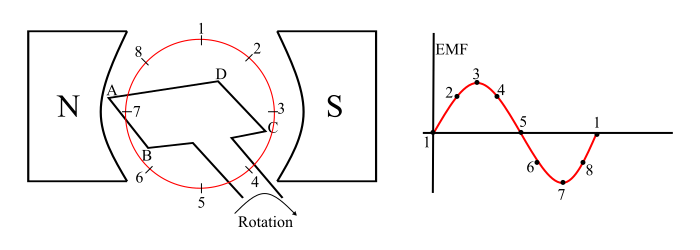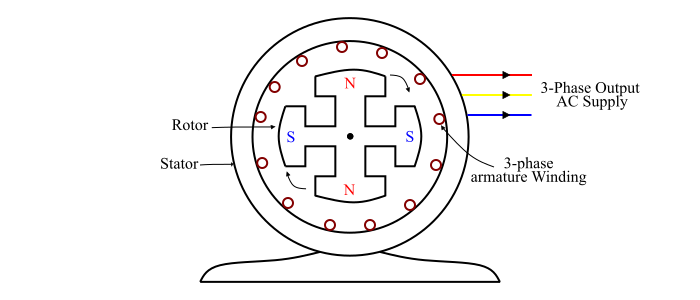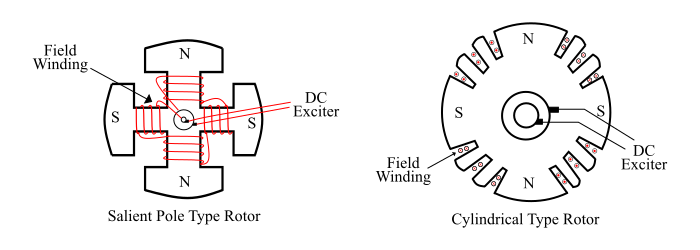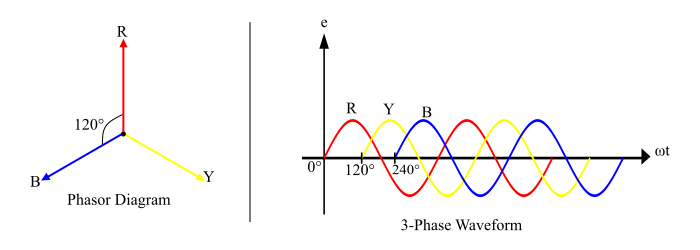# Types of AC Generators â€“ Single Phase and Three Phase AC Generator

AC generators are commonly known as synchronous generators or alternators which convert mechanical power into AC power. The term alternator is used for AC generator because it produces AC power. Also, it is called synchronous generator because it must be driven at a constant speed (equal to synchronous speed) to generate AC power of the desired frequency.

In general, two types of AC generators are used in practice −

• Single Phase AC Generator

• Three Phase AC Generator

## Single Phase AC Generator

A single phase AC generator is an electric machine that converts mechanical power into 1- phase electric power (AC) through the process of electromagnetic induction.

## Construction of Single Phase AC Generator

A single phase AC generator has following main parts −

• Magnetic Field System

• Armature Core

• Armature Winding

• Yoke## Magnetic Field System

The magnetic field system is used to produce uniform magnetic field in the single phase AC generator within which the armature rotates. The field system may be composed of either permanent magnets (small generators) or electromagnets (in large generators). Generally, the field system of a practical single phase AC generator consists of poles on which the field coils are mounted. When field coils are energised, a magnetic field produces within the generator.

## Armature Core

The armature core is mounted on the machine shaft and rotates in the magnetic field of field poles. It consists of slotted soft iron laminations that are stacked to form a cylindrical core. The laminations are insulated from each other by a thin film of varnish. The core is made laminated to reduce the eddy current loss. The armature conductors are placed into the slots, made on the outer periphery of the armature core.

## Armature Winding

The slots of the armature core hold insulated conductors, which are connected in a suitable manner. This is called as armature winding. This is the winding in which the working EMF induces.

## Yoke

The circular frame of the single phase AC generator is called yoke. The yoke of single phase AC generator is usually made of solid cast steel. The yoke increases the mechanical strength of the generator. It also provides path to the magnetic flux produced by the field winding.

## Working of Single Phase AC Generator

Consider a simple loop single phase AC generator (as shown in the figure), in this a single turn loop ‘ABCD’ is rotating clockwise in a uniform magnetic field with a constant speed. When the loop rotates, the magnetic flux linking the coil sides ‘AB’ and ‘CD’ changes continuously. This change in flux linkage induces an EMF in coil sides and the induced EMF in one coil side adds the induced EMF in the other.The EMF induced in a single phase AC generator can be explained as follows −

• When the loop is in position-1, the generated EMF is zero because, the movement of coil sides is parallel to the magnetic flux.

• When the loop is in position-2, the coil sides are moving at an angle to the magnetic flux and hence, a small EMF is generated.

• When the loop is in position-3, the coil sides are moving at right angle to the magnetic flux, therefore the generated EMF is maximum

• When the loop is in position-4, the coil sides are cutting the magnetic flux at an angle, thus a reduced EMF is generated in the coil sides.

• When the loop is in position-5, no flux linkage with the coil side and are moving parallel to the magnetic flux. Therefore, no EMF is generated in the coil.

• At the position-6, the coil sides move under a pole of opposite polarity and hence the polarity of generated EMF is reversed. The maximum EMF will generate in this direction at position-7 and zero when at position-1. This cycle repeats with revolution of the coil.

In this way, the alternating EMF is generated in a single phase AC generator, where the mechanical energy of rotation is converted into electrical energy.

## Three Phase AC Generator

A three phase AC generator is a synchronous machine that coverts mechanical power into three-phase AC power through the process of electromagnetic induction.

A three phase AC generator or alternator operates on the principle of electromagnetic induction i.e. when the magnetic flux linking a conductor or coil changes, an EMF is induced in the conductor or coil.

Important − A three phase AC generator has three identical armature windings displaced from each other by 120° electrical.

## Construction of Three Phase AC Generator

A three phase AC generator has 3-phase winding on the stator and a field winding excited by DC supply on the rotor.## Stator

The stator is the stationary part of the three phase AC generator and is built of sheet-steel laminations having slots on its inner periphery. A three phase armature winding is placed in the stator slots of the generator. The armature winding of a three phase AC generator is always connected in star and the neutral is connected to the earth.

## Rotor

The rotor of a three phase AC generator carries a field winding which is supplied by a DC source (called exciter). The DC source is generally, a small DC shunt or compound generator mounted on the shaft of the three phase AC generator. There are two types of rotor constructions being used in a three phase AC generator −

• Salient Pole Type Rotor (Used in low and medium speed (120-400 RPM) alternators)

• Cylindrical Type Rotor (Used in high speed (1500-3000 RPM) alternators)## Operation of Three Phase AC Generator

The rotor winding of the three phase AC generator is connected to a DC exciter, so that alternate N and S poles are developed on the rotor. When the rotor is rotated in anti-clockwise direction by a prime mover, the armature conductors are cut by the rotating magnetic field of rotor poles.

Consequently, an EMF is induced in the armature conductors due to electromagnetic induction. This induced EMF in the armature is alternating one because N and S poles of rotor alternatively pass the armature conductors. The direction of the induced EMF is given by Fleming’s right hand rule and the frequency is given by the equation,

$$\mathrm{f=\frac{N_{S}P}{120}\:\:HZ}$$

Where,

• $N_{S}$ = synchronous speed of rotating magnetic field = speed of rotor

• P = Number of rotor poles

The magnitude of induced EMF depends upon the speed of rotation and the DC excitation current. The magnitude of induced EMF in each phase of the armature winding would be the same but displaced by 120° electrical from each other (see the waveform and phasor diagram).The EMF equation of a three phase AC generator is given by,

$$\mathrm{E_{RMS}/Phase=2.22f\varphi Z\:Volts}$$

Since the armature winding of a three phase AC generator is star connected, thus the line voltage will be,

$$\mathrm{E_{L}=\sqrt{3}\times E_{ph}}$$